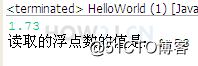# java零基础教学------操作符

2022年4月18日 53点热度 0人点赞 0条评论

 `+ - * / %`

 `++ --`

## 示例 1 : 基本算数操作符

 `+ - * /`

 `public` `class` `HelloWorld {` `    ``public` `static` `void` `main(String[] args) {` `        ``int` `i = ``10``;` `        ``int` `j = ``5``;` `        ``int` `a = i+j;` `        ``int` `b = i - j;` `        ``int` `c = i*j;` `        ``int` `d = i /j;` `    ``}` `}`

## 示例 2 : 任意运算单元的长度超过int

int a = 5;
long b = 6;
a+b -> 结果类型是long

 `public` `class` `HelloWorld {` `    ``public` `static` `void` `main(String[] args) {` `        ``int` `a = ``5``;` `        ``long` `b = ``6``;` `        ``int` `c = (``int``) (a+b); ``//a+b的运算结果是long型，所以要进行强制转换` `        ``long` `d = a+b;` `    ``}` `}`

## 示例 3 : 任意运算单元的长度小于int

byte a = 1;
byte b= 2;
a+b -> int 类型

 `public` `class` `HelloWorld {` `    ``public` `static` `void` `main(String[] args) {` `        ``byte` `a = ``1``;` `        ``byte` `b= ``2``;` `        ``byte` `c = (``byte``) (a+b); ``//虽然a b都是byte类型，但是运算结果是int类型，需要进行强制转换` `        ``int` `d = a+b;` `    ``}` `}`

## 示例 4 : %取模

% 取余数，又叫取模
5除以2，余1

 `public` `class` `HelloWorld {` `    ``public` `static` `void` `main(String[] args) {` `        ``int` `i = ``5``;` `        ``int` `j = ``2``;` `        ``System.out.println(i%j); ``//输出为1` `    ``}` `}`

## 示例 5 : 自增 自减

++
--

 `public` `class` `HelloWorld {` `    ``public` `static` `void` `main(String[] args) {` `        ``int` `i = ``5``;` `        ``i++;` `        ``System.out.println(i);``//输出为6` `    ``}` `}`

## 示例 6 : 自增 自减操作符置前以及置后的区别

int i = 5;
i++; 先取值，再运算
++i; 先运算，再取值

 `public` `class` `HelloWorld {` `    ``public` `static` `void` `main(String[] args) {` `        ``int` `i = ``5``;` `        ``System.out.println(i++); ``//输出5` `        ``System.out.println(i);   ``//输出6` `        ``int` `j = ``5``;` `        ``System.out.println(++j); ``//输出6` `        ``System.out.println(j);   ``//输出6` `    ``}` `}`

> 大于
>= 大于或等于
< 小于
<= 小于或等于
== 是否相等
!= 是否不等

## 示例 1 : 关系操作符

> 大于
>= 大于或等于
< 小于
<= 小于或等于
== 是否相等
!= 是否不等

 `public` `class` `HelloWorld {` `    ``public` `static` `void` `main(String[] args) {` `       ``int` `a = ``5``;` `       ``int` `b = ``6``;` `       ``int` `c = ``5``;` `       ``System.out.println(a>b);  ``//返回 false` `       ``System.out.println(a>=c);  ``//返回 true` `       ``System.out.println(a==b); ``//返回false` `       ``System.out.println(a!=b);``//返回true` `}` `}`

## 示例 1 : 长路与 和 短路与`public` `class` `HelloWorld {` `    ``public` `static` `void` `main(String[] args) {` `        ``//长路与  无论第一个表达式的值是true或者false,第二个的值，都会被运算` `        ``int` `i = ``2``;` `        ``System.out.println( i== ``1` `& i++ ==``2`  `); ``//无论如何i++都会被执行，所以i的值变成了3` `        ``System.out.println(i);` `        ``//短路与 只要第一个表达式的值是false的，第二个表达式的值，就不需要进行运算了` `        ``int` `j = ``2``;` `        ``System.out.println( j== ``1` `&& j++ ==``2`  `);  ``//因为j==1返回false,所以右边的j++就没有执行了，所以j的值，还是2` `        ``System.out.println(j);     ` `    ``}` `}`

## 示例 2 : 长路或 和 短路或`public` `class` `HelloWorld {` `    ``public` `static` `void` `main(String[] args) {` `        ``//长路或  无论第一个表达式的值是true或者false,第二个的值，都会被运算` `        ``int` `i = ``2``;` `        ``System.out.println( i== ``1` `| i++ ==``2`  `); ``//无论如何i++都会被执行，所以i的值变成了3` `        ``System.out.println(i);` `        ``//短路或 只要第一个表达式的值是true的，第二个表达式的值，就不需要进行运算了` `        ``int` `j = ``2``;` `        ``System.out.println( j== ``2` `|| j++ ==``2`  `);  ``//因为j==2返回true,所以右边的j++就没有执行了，所以j的值，还是2` `        ``System.out.println(j);     ` `    ``}` `}`

## 示例 3 : 取反

 `public` `class` `HelloWorld {` `    ``public` `static` `void` `main(String[] args) {` `        ``boolean` `b = ``true``;` `        ``System.out.println(b); ``//输出true` `        ``System.out.println(!b);``//输出false` `    ``}` `}`

## 示例 4 : 异或^`public` `class` `HelloWorld {` `    ``public` `static` `void` `main(String[] args) {` `        ``boolean` `a = ``true``;` `        ``boolean` `b = ``false``;` `        ``System.out.println(a^b); ``//不同返回真` `        ``System.out.println(a^!b); ``//相同返回假` `    ``}` `}`

## 示例 1 : 一个整数的二进制表达

 `public` `class` `HelloWorld {` `    ``public` `static` `void` `main(String[] args) {` `        ``int` `i = ``5``;` `        ``String b = (Integer.toBinaryString(i)); ``// 5的二进制的表达101` `        ``System.out.println(i+``" 的二进制表达是: "``+b);` `    ``}` `}`

## 示例 2 : 位或

5的二进制是101
6的二进制是110

 `public` `class` `HelloWorld {` `    ``public` `static` `void` `main(String[] args) {` `        ``int` `i  =``5``;` `        ``int` `j = ``6``;` `        ``System.out.println(Integer.toBinaryString(i)); ``//5的二进制是101` `        ``System.out.println(Integer.toBinaryString(j)); ``//6的二进制是110` `        ``System.out.println(i|j); ``//所以 5|6 对每一位进行或运算，得到 111->7` `    ``}` `}`

## 示例 3 : 位与

5的二进制是101
6的二进制是110

 `public` `class` `HelloWorld {` `    ``public` `static` `void` `main(String[] args) {` `        ``int` `i  =``5``;` `        ``int` `j = ``6``;` `        ``System.out.println(Integer.toBinaryString(i)); ``//5的二进制是101` `        ``System.out.println(Integer.toBinaryString(j)); ``//6的二进制是110` `        ``System.out.println(i&j); ``//所以 5&6 对每一位进行与运算，得到 100->4` `    ``}` `}`

## 示例 4 : 异或

5的二进制是101
6的二进制是110

 `public` `class` `HelloWorld {` `    ``public` `static` `void` `main(String[] args) {` `        ``int` `i  =``5``;` `        ``int` `j = ``6``;` `        ``System.out.println(Integer.toBinaryString(i)); ``//5的二进制是 101` `        ``System.out.println(Integer.toBinaryString(j)); ``//6的二进制是110` `        ``System.out.println(i^j); ``//所以 5^6 对每一位进行或运算，得到 011->3` `        ``System.out.println(i^``0``);` `        ``System.out.println(i^i);` `    ``}` `}`

## 示例 5 : 取非

5 的二进制是 00000101

 `public` `class` `HelloWorld {` `    ``public` `static` `void` `main(String[] args) {` `        ``byte` `i  =``5``;` `        ``System.out.println(Integer.toBinaryString(i)); ``//5的二进制是00000101,所以取非即为11111010,即为-6` `        ``System.out.println(~i);` `    ``}` `}`

## 示例 6 : 左移 右移

 `public` `class` `HelloWorld {` `    ``public` `static` `void` `main(String[] args) {` `        ``byte` `i  =``6``;` `        ``//6的二进制是110` `        ``System.out.println(Integer.toBinaryString(i));` `        ``//6向左移1位后，变成1100，对应的10进制是12` `        ``System.out.println(i<<``1``);` `        ``//6向右移1位后，变成11，对应的10进制是3` `        ``System.out.println(i>>``1``);` `    ``}` `}`

## 示例 7: 带符号右移与无符号右移

 `public` `class` `HelloWorld {` `    ``public` `static` `void` `main(String[] args) {` `        ``int` `i  =-``10``;` `        ``//-10的二进制是11111111111111111111111111110110` `        ``//第一位是1，即符号位，代表这是一个负数` `        ``System.out.println(Integer.toBinaryString(i));` `        ``//对于正数， 带符号右移 >> 会把所有的位右移，并在最前面补0` `        ``//对于负数， 带符号右移 >> 会把所有的位右移，并在最前面补1` `        ``//-10带符号右移1位，移动后前面补齐1` `        ``//得到11111111111111111111111111111011` `        ``//因为第一位是1，所以依然是一个负数，对应的十进制是-5` `        ``int` `j = i>>``1``;` `        ``System.out.println(Integer.toBinaryString(j));` `        ``System.out.println(j);` `        ``//-10无符号向右移1位，符号位也会向右移，第一位就变成了0` `        ``//得到01111111111111111111111111111011，对应的十进制是2147483643` `        ``int` `k = i>>>``1``;` `        ``System.out.println(Integer.toBinaryString(k));     ` `        ``System.out.println(k);` `    ``}` `}`

## 示例 1 : 赋值操作

int i = 5+5;

 `public` `class` `HelloWorld {` `    ``public` `static` `void` `main(String[] args) {` `        ``int` `i = ``5``+``5``;` `    ``}` `}`

## 示例 2 : 对本身进行运算，并赋值

+=即自加
i+=2;

i=i+2;

 `public` `class` `HelloWorld {` `    ``public` `static` `void` `main(String[] args) {` `        ``int` `i =``3``;` `        ``i+=``2``;` `        ``System.out.println(i);` `        ``int` `j=``3``;` `        ``j=j+``2``;` `        ``System.out.println(j);     ` `    ``}` `}`

?:

## 示例 1 : 三元操作符

 `public` `class` `HelloWorld {` `    ``public` `static` `void` `main(String[] args) {` `        ``int` `i = ``5``;` `        ``int` `j = ``6``;` `        ``int` `k = i < j ? ``99` `: ``88``;` `        ``// 相当于` `        ``if` `(i < j) {` `            ``k = ``99``;` `        ``} ``else` `{` `            ``k = ``88``;` `        ``}` `        ``System.out.println(k);` `    ``}` `}`

## 步骤 1 : 使用Scanner读取整数

 `import` `java.util.Scanner;`

 `import` `java.util.Scanner;` `public` `class` `HelloWorld {` `    ``public` `static` `void` `main(String[] args) {` `        ``Scanner s = ``new` `Scanner(System.in);` `        ``int` `a = s.nextInt();` `        ``System.out.println(``"第一个整数："``+a);` `        ``int` `b = s.nextInt();` `        ``System.out.println(``"第二个整数："``+b);` `    ``}` `}`

## 步骤 2 : 使用Scanner读取浮点数`import` `java.util.Scanner;` `public` `class` `HelloWorld {` `    ``public` `static` `void` `main(String[] args) {` `        ``Scanner s = ``new` `Scanner(System.in);` `        ``float` `a = s.nextFloat();` `        ``System.out.println(``"读取的浮点数的值是："``+a);` `    ``}` `}`

## 步骤 3 : 使用Scanner读取字符串

 `import` `java.util.Scanner;` `public` `class` `HelloWorld {` `    ``public` `static` `void` `main(String[] args) {` `        ``Scanner s = ``new` `Scanner(System.in);` `        ``String a = s.nextLine();` `        ``System.out.println(``"读取的字符串是："``+a);` `    ``}` `}`

## 步骤 4 : 读取了整数后，接着读取字符串

 `import` `java.util.Scanner;` `public` `class` `HelloWorld {` `    ``public` `static` `void` `main(String[] args) {` `        ``Scanner s = ``new` `Scanner(System.in);` `        ``int` `i = s.nextInt();` `        ``System.out.println(``"读取的整数是"``+ i);` `        ``String rn = s.nextLine();` `        ``String a = s.nextLine();` `        ``System.out.println(``"读取的字符串是："``+a);` `    ``}` `}`zdc1228@163.com